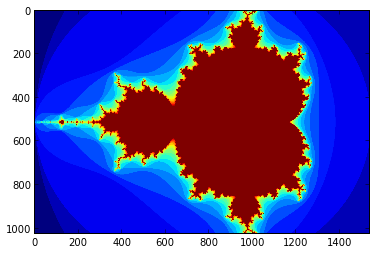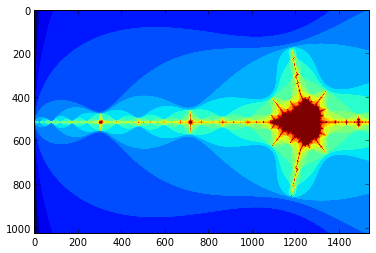# A NumbaPro Mandelbrot Example¶

This notebook was written by Mark Harris based on code examples from Continuum Analytics that I modified somewhat. This is an example that demonstrates accelerating a Mandelbrot fractal computation using "CUDA Python" with NumbaPro.

Let's start with a basic Python Mandelbrot set. We use a numpy array for the image and display it using pylab imshow.

In :
import numpy as np
from pylab import imshow, show
from timeit import default_timer as timer


The mandel function performs the Mandelbrot set calculation for a given (x,y) position on the imaginary plane. It returns the number of iterations before the computation "escapes".

In :
def mandel(x, y, max_iters):
"""
Given the real and imaginary parts of a complex number,
determine if it is a candidate for membership in the Mandelbrot
set given a fixed number of iterations.
"""
c = complex(x, y)
z = 0.0j
for i in range(max_iters):
z = z*z + c
if (z.real*z.real + z.imag*z.imag) >= 4:
return i

return max_iters


create_fractal iterates over all the pixels in the image, computing the complex coordinates from the pixel coordinates, and calls the mandel function at each pixel. The return value of mandel is used to color the pixel.

In :
def create_fractal(min_x, max_x, min_y, max_y, image, iters):
height = image.shape
width = image.shape

pixel_size_x = (max_x - min_x) / width
pixel_size_y = (max_y - min_y) / height

for x in range(width):
real = min_x + x * pixel_size_x
for y in range(height):
imag = min_y + y * pixel_size_y
color = mandel(real, imag, iters)
image[y, x] = color


Next we create a 1024x1024 pixel image as a numpy array of bytes. We then call create_fractal with appropriate coordinates to fit the whole mandelbrot set.

In :
image = np.zeros((1024, 1536), dtype = np.uint8)
start = timer()
create_fractal(-2.0, 1.0, -1.0, 1.0, image, 20)
dt = timer() - start

print "Mandelbrot created in %f s" % dt
imshow(image)
show()

Mandelbrot created in 6.921606 sYou can play with the coordinates to zoom in on different regions in the fractal.

In :
create_fractal(-2.0, -1.7, -0.1, 0.1, image, 20)
imshow(image)
show()## Faster Execution with Numba¶

Numba is a Numpy-aware dynamic Python compiler based on the popular LLVM compiler infrastructure.

Numba is an Open Source NumPy-aware optimizing compiler for Python sponsored by Continuum Analytics, Inc. It uses the remarkable compiler infrastructure to compile Python syntax to machine code. It is aware of NumPy arrays as typed memory regions and so can speed-up code using NumPy arrays, such as our Mandelbrot functions.

The simplest way to use Numba is to decorate the functions you want to compile with @autojit. Numba will compile them for the CPU (if it can resolve the types used).

In :
from numba import autojit

@autojit
def mandel(x, y, max_iters):
"""
Given the real and imaginary parts of a complex number,
determine if it is a candidate for membership in the Mandelbrot
set given a fixed number of iterations.
"""
c = complex(x, y)
z = 0.0j
for i in range(max_iters):
z = z*z + c
if (z.real*z.real + z.imag*z.imag) >= 4:
return i

return max_iters

@autojit
def create_fractal(min_x, max_x, min_y, max_y, image, iters):
height = image.shape
width = image.shape

pixel_size_x = (max_x - min_x) / width
pixel_size_y = (max_y - min_y) / height

for x in range(width):
real = min_x + x * pixel_size_x
for y in range(height):
imag = min_y + y * pixel_size_y
color = mandel(real, imag, iters)
image[y, x] = color


Let's run the @autojit code and see if it is faster.

In :
image = np.zeros((1024, 1536), dtype = np.uint8)
start = timer()
create_fractal(-2.0, 1.0, -1.0, 1.0, image, 20)
dt = timer() - start

print "Mandelbrot created in %f s" % dt
imshow(image)
show()

Mandelbrot created in 0.065591 sOn my desktop computer, the time to compute the 1024x1024 mandelbrot set dropped from 6.92s down to 0.06s. That's a speedup of 115x! The reason this is so much faster is that Numba uses Numpy type information to convert the dynamic Python code into statically compiled machine code, which is many times faster to execute than dynamically typed, interpreted Python code.

## Even Bigger Speedups with CUDA Python¶

Anaconda, from Continuum Analytics, is a "completely free enterprise-ready Python distribution for large-scale data processing, predictive analytics, and scientific computing." Anaconda Accelerate is an add-on for Anaconda that includes the NumbaPro Python compiler.

NumbaPro is an enhanced Numba that targets multi-core CPUs and GPUs directly from simple Python syntax, providing the performance of compiled parallel code with the productivity of the Python language.

## CUDA Python¶

In addition to various types of automatic vectorization and generalized Numpy Ufuncs, NumbaPro also enables developers to access the CUDA parallel programming model using Python syntax. With CUDA Python, you use parallelism explicitly just as in other CUDA languages such as CUDA C and CUDA Fortran.

Let's write a CUDA version of our Python Mandelbrot set. We need to import cuda from the numbapro module. Then, we need to create a version of the mandel function compiled for the GPU. We can do this without any code duplication by calling cuda.jit on the function, providing it with the return type and the argument types, and specifying device=True to indicate that this is a function that will run on the GPU device.

In :
from numbapro import cuda
from numba import *

mandel_gpu = cuda.jit(restype=uint32, argtypes=[f8, f8, uint32], device=True)(mandel)


In CUDA, a kernel is a function that runs in parallel using many threads on the device. We can write a kernel version of our mandelbrot function by simply assuming that it will be run by a grid of threads. NumbaPro provides the familiar CUDA threadIdx, blockIdx, blockDim and gridDim intrinsics, as well as a grid() convenience function which evaluates to blockDim * blockIdx + threadIdx.

Our example juse needs a minor modification to compute a grid-size stride for the x and y ranges, since we will have many threads running in parallel. We just add these three lines:

startX, startY = cuda.grid(2)
gridX = cuda.gridDim.x * cuda.blockDim.x;
gridY = cuda.gridDim.y * cuda.blockDim.y;



And we modify the range in the x loop to use range(startX, width, gridX) (and likewise for the y loop).

We decorate the function with @cuda.jit, passing it the type signature of the function. Since kernels cannot have a return value, we do not need the restype argument.

In :
@cuda.jit(argtypes=[f8, f8, f8, f8, uint8[:,:], uint32])
def mandel_kernel(min_x, max_x, min_y, max_y, image, iters):
height = image.shape
width = image.shape

pixel_size_x = (max_x - min_x) / width
pixel_size_y = (max_y - min_y) / height

startX, startY = cuda.grid(2)
gridX = cuda.gridDim.x * cuda.blockDim.x;
gridY = cuda.gridDim.y * cuda.blockDim.y;

for x in range(startX, width, gridX):
real = min_x + x * pixel_size_x
for y in range(startY, height, gridY):
imag = min_y + y * pixel_size_y
image[y, x] = mandel_gpu(real, imag, iters)


#### Device Memory¶

CUDA kernels must operate on data allocated on the device. NumbaPro provides the cuda.to_device() function to copy a Numpy array to the GPU.

d_image = cuda.to_device(image)



The return value (d_image) is of type DeviceNDArray, which is a subclass of numpy.ndarray, and provides the to_host() function to copy the array back from GPU to CPU memory

d_image.to_host()

#### Launching Kernels¶

To launch a kernel on the GPU, we must configure it, specifying the size of the grid in blocks, and the size of each thread block. For a 2D image calculation like the Mandelbrot set, we use a 2D grid of 2D blocks. We'll use blocks of 32x8 threads, and launch 32x16 of them in a 2D grid so that we have plenty of blocks to occupy all of the multiprocessors on the GPU.

Putting this all together, we launch the kernel like this.

In :
gimage = np.zeros((1024, 1536), dtype = np.uint8)
blockdim = (32, 8)
griddim = (32,16)

start = timer()
d_image = cuda.to_device(gimage)
mandel_kernel[griddim, blockdim](-2.0, 1.0, -1.0, 1.0, d_image, 20)
d_image.to_host()
dt = timer() - start

print "Mandelbrot created on GPU in %f s" % dt

imshow(gimage)
show()

Mandelbrot created on GPU in 0.003409 sYou may notice that when you ran the above code, the image was generated almost instantly. On the NVIDIA Tesla K20c GPU installed in my desktop, it ran in 311 milliseconds, which is an additional 19.3x speedup over the @autojit (compiled CPU) code, or a total of over 2000x faster than interpreted Python code.